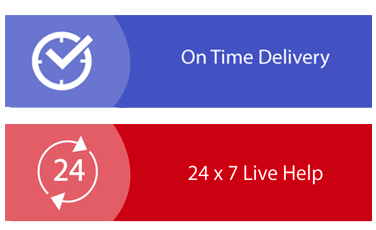× Limited Time Offer ! FLAT 30-40% off - Grab Deal Before It’s Gone. Order Now

## STAT6000 Statistics for Public Health Assignment Help

### Assignment Brief

Length 2000
Learning Outcomes:

This assessment addresses the following learning outcomes:

1. Understand key concepts in statistics and the way in which both descriptive and inferential statistics are used to measure, describe and predict health and illness and the effects of interventions.

2. Apply key terms and concepts of statistics for assignment help including; sampling, hypothesis testing, validity and reliability, statistical significance and effect size.

3. Interpret the results of commonly used statistical tests presented in published literature.

Submission Due Sunday following the end of Module 4 at 11:55pm AEST/AEDT*

Weighting - 30%
Total Marks - 100 marks

### Instructions:

This assessment requires you to read two articles and answer a series of questions in no more than 2000 words. Most public health and wider health science journals report some form of statistics. The ability to understand and extract meaning from journal articles, and the ability to critically evaluate the statistics reported in research papers are fundamental skills in public health.

Read the Riordan, Flett, Hunter, Scarf and Conner (2015) research article and answer the following
questions:

1. This paper presents two hypotheses. State the null and alternative hypothesis for each one, and describe the independent and dependent variables for each hypothesis.

2. What kind of sampling method did they use, and what are the advantages and disadvantages of recruiting participants in this way?

3. What are the demographic characteristics of the people in the sample? Explain by referring to the descriptive statistics reported in the paper.

4. What inferential statistics were used to analyze data in this study, and why?

5. Regarding the relationship between FoMO scores, weekly drinks, drinking frequency, drinking quantity, and BYAACQ. Answer the following questions;

a) Which variable had the weakest association with FoMO score?

b) Which variable had the strongest association?

c) Was the association (weakest and strongest) statistically significant?

d) What are the correlation coefficients for both associations (weakest and strongest)?

e) State how much variation in weekly drinks, drinking frequency, drinking quantity, and BYAAC is attributed toFoMO scores.

f) What variables are controlled in the correlation analysis test?

6. How representative do you think the sample is of the wider population of college students in New Zealand? Explain why.

Paper 2: Wong, M. C., S., Leung, M. C., M., Tsang, C. S., H., . . . Griffiths, S. M. (2013). The rising tide
of diabetes mellitus in a Chinese population: A population-based household survey on 121,895
http://dx.doi.org.ezproxy.laureate.net.au/10.1007/s00038-012-0364-y

Read the Wong et. al. (2014) paper and answer the following questions:

1. Describe the aims of the study. Can either aim be restated in terms of null and alternative hypotheses? Describe these where possible.

2. What are the demographic characteristics of the people in the sample? Explain by referring to the descriptive statistics reported in the paper.

3. What inferential statistics were used to analyze data in this paper, and why?

4. What did the researchers find when they adjusted the prevalence rates of diabetes for age and sex?

5. Interpret the odds ratios for self-reported diabetes diagnosis to explain who is at the greatest risk of diabetes.

6. What impact do the limitations described by the researchers have on the extent to which the results can be trusted, and why?

### Assessment Criteria

• Knowledge of sampling methods, and research and statistical concepts 20%

• Interpretation of research concepts, statistical concepts and reported results, demonstrating applied knowledge and understanding 40 %

• Critical analysis of research elements including sampling, results and limitations 30%

• Academic writing (clarity of expression, correct grammar and punctuation, correct word use) and accurate use of APA referencing style 10%

## Solution

Riordan, Flett, Hunter, Scarf and Conner (2015) research article answers:

###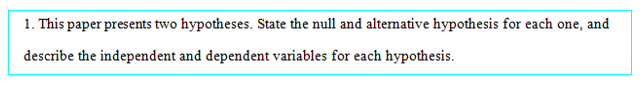Hypothesis 1:

Null hypothesis: H0: Students’ alcohol consumption frequency was not dependent on FoMO score.

Alternate hypothesis: HA: Students’ with higher FoMO scores consumed increased amount of alcohol compared to those with lower FoMO scores.

Independent Variable: Alcohol consumption frequency of the participants of the study was considered assignment writing-point psychometric scale FoMO (“Fear of missing out”) was considered as the dependent variable. A variation between prevalent apprehensions of the participants regarding engagement in social engagements was measured using the FoMO.

Hypothesis 2:

Null hypothesis: H0: There was no relation between FoMO score and alcohol-related consequences.

Alternate hypothesis: HA: Students with higher FoMO score will come across more alcohol-related consequences compared to those with lower in FoMO.

Independent Variable: Alcohol related consequences measured using B-YAACQ scale, which assessed negative impacts of alcohol drinking for last three months.

Dependent Variable: The 10-point psychometric scale FoMO (“Fear of missing out”) was considered as the dependent variable.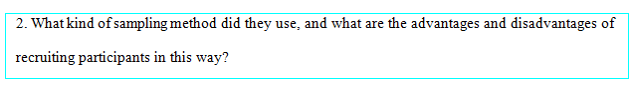Sampling Technique: The research analyzed two studies where data was collected from the University of Otago, Dunedin. The first study was a cross sectional study where data from 182 students was collected in a convenience sampling methodology. Study 2 had a research methodology of ‘daily diary study’, where 262 participants were recruited from psychology classes.

Advantage: Convenience samples are an economical way of collecting data. It doesn't take much effort and money for initiate a convenience sampling methodology. As, in the present study survey link was posted on a departmental page where students can vote online. Therefore, it is one of the most economical options for the collection of data in the study that also saves time while gathering information. This is also useful as an intervention to collect feedback from hesitant participants as one can contact people about specific questions related to the study within minutes while using this method. Surveys can get partners to help provide more information about a person's demographic profile so that normalization can be created in a large group in the future.

Disadvantage: Information received from the study using convenient sampling may not represent characteristics of the general population. Therefore, conclusions based on the collected data may not provide information about the entire Otage population. Moreover, it was difficult to know whether some participants provided incorrect information or not. In future studies, it also becomes difficult to replicate the results due to nature of the collected data from the convenience sampling. Again, such data collected fails to show differences that may exist between multiple subgroups is one of the limitations of the present study which fails to differentiate between FoMO scores of men and women.

###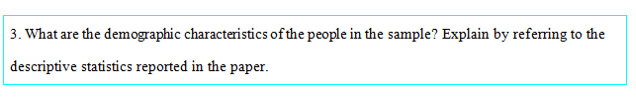Age, gender, and ethnicity are the three demographic characteristic details available in the paper. Explanation: Among 182 participants in the study 1, 78.6% were female participants. All of the study subjects were aged between 18-25 years with an average age of 19.4 years and a standard deviation of 1.4 years. Ethnicity wise categorization revealed that the sample was predominantly New Zealand European origin with presence of 80.8%. Rest of them was Asian (3.8%), Maori or Pacific Islander (6.0%), or belonged to other (7.7%) ethnic groups. A larger sample of 262 students participated in study 2, where 75.3% were female. The age bracket was 18-25 years with average of 19.6 years and a standard deviation of 1.6 years. Predominant presence of New Zealand European descent was noted (76%), where 12.2% were Asian, 7.2% were Maori or Pacific Islander, and 4.6% from other ethnicities.

###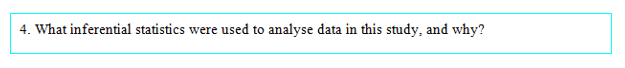Inferential Tests: Two inferential statistics were used for testing the hypotheses. An independent t-test was administered to compare frequency of alcohol consumption between men and women. Alongside, Pearson’s correlation test was used to assess the relation between FoMO scores, alcohol consumption frequency and negative effects of alcohol consumption measured with B-YAACQ scale.

Reason of Use: An independent t-test was used to compare average drinking frequencies between male and female students by comparing their average drinking frequencies together with the standard deviations.

Pearson’s correlation coefficient was used to find the pairwise relation between FoMOs mean, weekly frequency of drinks, drinking quantity, drinking frequency, and B-YAACQ scale (Riordan et al., 2015).

###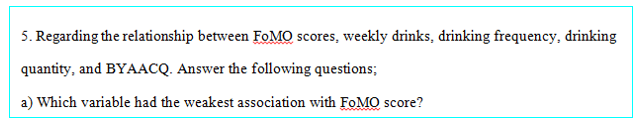In study 1, weekly drinks had the weakest relation with FoMO score. In study 2, drinking frequency had the weakest relation with FoMO score.

###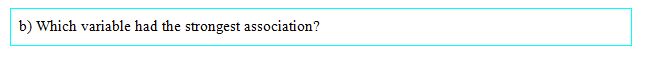In both the studies, B-YAACQ scale score had the strongest relationship with FoMO score.

###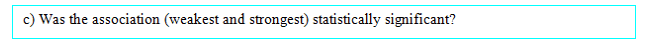The weakest associations were not statistically significant, whereas the strongest relationship between B-YAACQ scale score and FoMO score was statistically significant.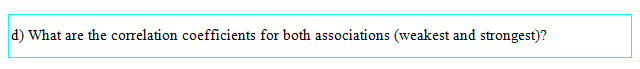Study 1:

The correlation coefficient between Weekly drinks with FoMO score was -0.014 (weakest)

The correlation coefficient between B-YAACQ scale score and FoMO score was 0.249 (strongest)

Study 2:

The correlation coefficient between drinking frequency with FoMO score was 0.092 (weakest)
The correlation coefficient between B-YAACQ scale score and FoMO score was 0.301(strongest)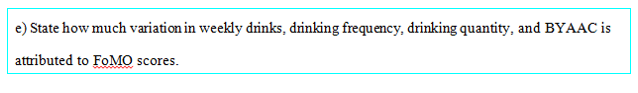Overall, FoMOs score was not associated to the amount and frequency of weekly consumption of alcohol. In Study 1, there was no link between the average amount of FoMOs and alcohol. However, in study 2, there was a significant association between drinking session quantity and the FoMOs scores. FoMO scores impacted drinking session quantity with a 2.8% variance in Study 2, corresponding to Cohen's d ("small" effect) of 0.339. In addition, in both studies, association of FoMOs with alcohol-related higher number of severe negative consequences over the past three months is also a major concern. In both the studies, the amount negative alcohol outcomes varied by 6.2% and 9.1% was due to FoMOs, corresponding to 0.514 and 0.631 Cohen d (moderate effects).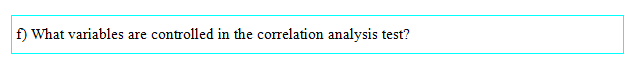Age and gender of the participants were the two controlled variables in the correlation analysis.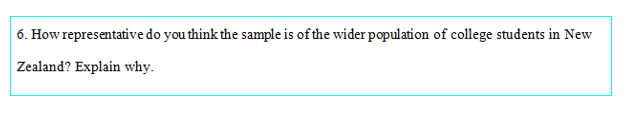All the participants belonged to the age group of 18-25 years that indeed can represent wider undergraduate population of New Zealand universities. However, the age group seems inadequate to represent graduate students from universities.

Reason:

The experimental data were collected from undergraduate college students of the University of Otago, Dunedin (New Zealand). The first study used cross-sectional study with convenience sampling to include 182 students as participants, and the second study went with daily diary study including 262 participants. The convenience sampling technique used to collect data also indicates possible presence of falsified data. Hence, sample of the present study is representative of wider undergraduate population of colleges in New Zealand. However, the wider representation of all the students from the entire nation seems not possible using the sample of this study.

### Wong et al (2013) research article answers: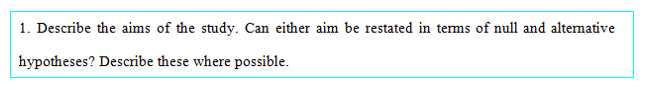Primary objective of the studied paper was to assess the generality of results found from analysing the effect of age, household income, and sex on diabetes prevalence among 121,895 participants representing entire Hong Kong population. The survey was conducted in 2001, 2002, 2005, and 2008 to evaluate results across a period of 8 years. The entire sample was stratified in two strata based on gender of the participants (Wong et al., 2013).

First Objective was to assess the effect of increase in age on diabetes prevalence among the participants.

Null hypothesis: H0: There existed no association between increase in age and diabetes prevalence.

Alternate hypothesis: H0: There existed statistically significant association between increase in age and diabetes prevalence (0-39 was referent age group).

Second Objective was to assess the effect of low household income on diabetes prevalence among the participants.

Null hypothesis: H0: There existed no association between low household income and diabetes prevalence.
Alternate hypothesis: H0: There existed statistically significant association between low household income and diabetes prevalence (participants earning above \$ 50,000 referent income group).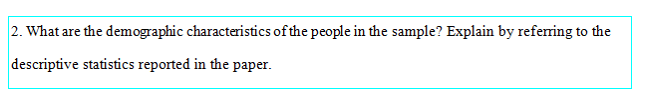Diabetes prevalence of 121,895 people across 2001, 2002, 2005, and 2008 was collected with demographic information regarding their age, household income, and gender. The sample consisted of 103,367 adult participants with age of 15 years and more. The average age of participants in the sample was calculated to be 38.2 years.

Information on gender of 121,895 participants revealed a balances presence of both the genders with females (N = 61, 831, 50.2%) being just greater in number. Household income (HK dollars) of sample participants was categorised in four categories (≥ 50,000, 25,000-49,999, 10,000-24,999, and ≤ 9,999). Presence of 10,000-24,999 income group of participants was the highest (N = 50,648, 42.4%), followed by 10,000-24,999 income group (N = 32,748, 27.4%), ≤ 9,999 (N = 23,578, 19.7%), and ≥ 50,000 (N = 12,452, 10.4%).

Sample was categorized according to age (years) in eight groups (< 15, 15-24, 25-34, 35-44, 45-54, 55-64, 65-74, and ≥ 75). Among 103,367 adult participants (≥ 15), 13.8% (N = 16, 834) belonged to age group of 15-24, 14.6% (N = 17,751) to age group of 25-34, 18.2% (N = 22,206) to age group of 35-44, 16.4% (N = 20,033) to age group of 15-24, 9.2% (N = 11,179) to age group of 15-24, and a total of 12.6% (N = 15,364) belonged to age groups of 65-74, and ≥ 75.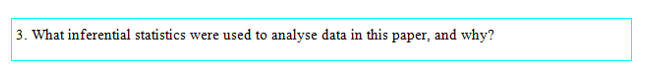Inferential analysis for evaluating the impact of age and income on diabetes prevalence across years was Binary Logistic Regression. In the constructed model age and groups were adjusted for better comparison. The age group of 0-39 years was the referent, whereas income group of ‘≥ 50,000’ was considered as the referent in the regression model. A multivariate regression model was also used to assess the independent association between diabetes prevalence and participants’ demographic details.

Initially, use of multivariate regression model indicated the causal relation and association between diabetes and demographic factors. Binary Logistic Regression models are generally used where the dependent variable has two categories. The linear regression model fails to assess the impact of predictors on two different categories of an outcome variable. The odd ratios in the Binary Logistic regression models display the exact relation with the predictor, especially with reference to age and income referent categories.

The results in the Binary Logistic Regression model were statistically significant when age and sex were adjusted for measuring diabetes prevalence. Two separate regression models were constructed based on gender, and in each model age groups were reorganized to better comparison of diabetes prevalence. Importantly, the study also considered 2001 as base year or referent year to compare the results of 2005 and 2008.

Initially, females were noted to be (31.8%, 2005; 69.3%, 2008) have higher diabetes prevalence compared to that of the males (27.8%, 2005; 47.9%, 2008). But, when adjusted for sex no significant difference in diabetes prevalence was noted between male and females. Also, significantly increasing diabetes prevalence was noted for lower household income group when compared to highest income group.

Adjusted Odd Ratio (AOR) for sex and age were evaluated from the Logistic Regression Model. Age adjusted groups comparison revealed that people aged between 40 and 65 years (AOR = 32.21, 95% CI 20.6–50.4, p < 0.001) were significantly at higher risk of diabetes prevalence compared to the referent age group of 0-39 years. Notably, people aged over 65 years were 120 times more associated (AOR = 120.1, 95% CI 76.6–188.3, p < 0.001) to diabetes compared to referent group.

Monthly household income category of 25,000-49,999 (AOR = 1.39, 95% CI 1.04-1.86, p < 0.05), 10,000-24,999 (AOR = 1.58, 95% CI 1.2-2.07, p < 0.001), and ≤ 9,999 (AOR = 2.19, 95% CI 1.66-2.88, p < 0.001) were all significantly at a higher risk of association with diabetes compared to highest income group (≥ 50,000), especially the lowest income group had almost two-fold chance of diabetes in such comparison.

The coefficient of determination in the Binary Logistic Regression model was R2 = 0.198, implying that adjusted variables were able to explain 19.8% variation in diabetes prevalence. Hence, search of other predictors of diabetes prevalence, such as eating habit, family history, and affinity towards sugar and carb would have been beneficial.

Also, it has to be noted that the sample data was collected from self-reported survey of Chinese people. From previous literatures, it can be illustrated that most of the people in China are ignorant about preventive diabetes check-up (Yang et. al., 2010). Therefore, the self-reported data could have been erroneous and skewed. Generalization of the statistical analyses of the study could be a terrible mistake.

### References

Riordan, B. C., Flett, J. A., Hunter, J. A., Scarf, D., & Conner, T. S. (2015). Fear of missing out (FoMO): The relationship between FoMO, alcohol use, and alcohol-related consequences in college students. Annals of Neuroscience and Psychology, 2(7), 1-7.

Wong, M. C., Leung, M. C., Tsang, C. S., Lo, S. V., & Griffiths, S. M. (2013). The rising tide of diabetes mellitus in a Chinese population: a population-based household survey on 121,895 persons. International journal of public health, 58(2), 269-276.

Yang, W., Lu, J., Weng, J., Jia, W., Ji, L., Xiao, J., ... & Zhu, D. (2010). Prevalence of diabetes among men and women in China. New England Journal of Medicine, 362(12), 1090-1101.

## BEO1106 Business Statistics Assignment Sample

### Introduction

The price of a property can be determined by a number of factors (in addition to the market
trend). These factors may include (but not the least): The location, the land size, the size of the built area, the building type, the property type, number of rooms, number of bathroom and toilets, swimming pool, tennis court and so on.

The sample data you collected for your assignment contain the following variables:

V1 = Region where property is located (1 = North, 2 = West, 3 = East, 4 = Central)
V2 = Property type (0 = Unit, 1 = House)
V3 = Sale result (1 = Sold at auction, 2 = Passed-in, 3 = Private sale, 4 = Sold before auction).
Note that a blank cell for this variable indicates that the property did not sell.
V4 = Building type (1 = Brick, 2 = Brick veneer, 3 = Weatherboard, 4 = Vacant land)
V5 = Number of rooms
V6 = Land size (Square meters)
V7 = Sold Price (\$000s)

### Requirement

In relation to the Simple Regression topic of Business Statistics, for this Case Study, you are
required to conduct a regression analysis to estimate the relation between Number of Rooms and Advertised Price of properties in Melbourne.

### Instruction

You need to prepare a sample data using the Number of Rooms and the Advertised Price variables. You may find that V5 (Number of Rooms) variable has some missing observations in your sample. In order for Excel to estimate a regression equation, Excel requires a balanced data set. This means that both dependent variables and independent variables must have the same (balanced) number of observations in the data set. To balance the data set, we have to remove the observations which contain missing data. Refer to the steps in the Excel file Regression Estimation example for Case Study.xlsx to assist you to construct your balanced sample data set for the regression analysis.

In the Answer Sheet provided, name the dependent variable (Y) and the independent variable (X). Provide a brief explanation for assignment help to support your choice.

In a sentence, explain whether you expect a positive or a negative relation between the X and the Y variables.

Use Excel to produce a scatterplot using the independent variable for the horizontal (X) axis and the dependent variable as the vertical (Y) axis. Copy and paste the scatterplot to the Answer Booklet.

Hint: Follow the graph presentation (in Step 5, Regression Estimation example for Case
Study.xlsx).

Note:Title of the scatterplot and the labels for axes will account for 0.5 mark for each.

Follow the Excel procedure (select Data / Data Analysis / Regression) outlined on seminar note Slide 16, using the X variable and the Y variable you nominated in Task 1, generate regression estimation output tables. Copy the Regression Statistics and Coefficients tables (refer to Slide 27 and Slide 28) to the Answer Booklet.

Refer to the Regression Statistics table in Task 4, briefly describe the strength of the correlation between X and Y variables. Ensure your statement is supported by the statistic figure from the table.

Does the information shown in the Coefficients table agree with your expectation in Task 2?

Refer to the Coefficients table, and follow the presentation on seminar note Slide 19, construct the least squares linear regression equation for the relationship between the independent variable and the dependent variable.

Interpret the estimated intercept and the slope coefficients.

Select one of the two following scenarios which describe your choice in Task 1.

• In Task 1, if you nominated Number of Rooms is the independent variable, then you are asked to estimate the Advertised Price (dependent variable) of a property given the number of rooms of the property is 5.

• In Task 1, if you nominated Advertised Price is the independent variable, then you are asked to estimate the Number of Rooms (dependent variable) of a property given the advertised price is \$1.55 (million).

With reference to the R Square value provided in the Regression Statistics table, explain whether you would trust your estimation in Task 9. Comment on whether your answer in Task10 agrees with the answer in Task 5 in terms of the strength of the linear relationship between X and Y.

State, symbolically, the null and alternative hypotheses for testing whether there is a positive linear relationship between Number of Rooms and Advertised Price in the population.

Use the Empirical Rule, state the z- value which is corresponding to 2.5% significant level.

Use the p-value approach to decide, at a 2.5% level of significance, whether the null hypothesis of the test referred to in Task 11 can be rejected (or not). Make sure you provide a justification for your decision.

Following the decision in Task13, provide a precise conclusion to the hypothesis test conducted in Task 13.

From information provided in the Coefficients table, construct a 95% confidence interval estimate of the gradient of the population regression line. Is this interval consistent with the conclusion to the hypothesis test you arrived at in Task 14? Briefly explain the reasoning behind your answer

## Solution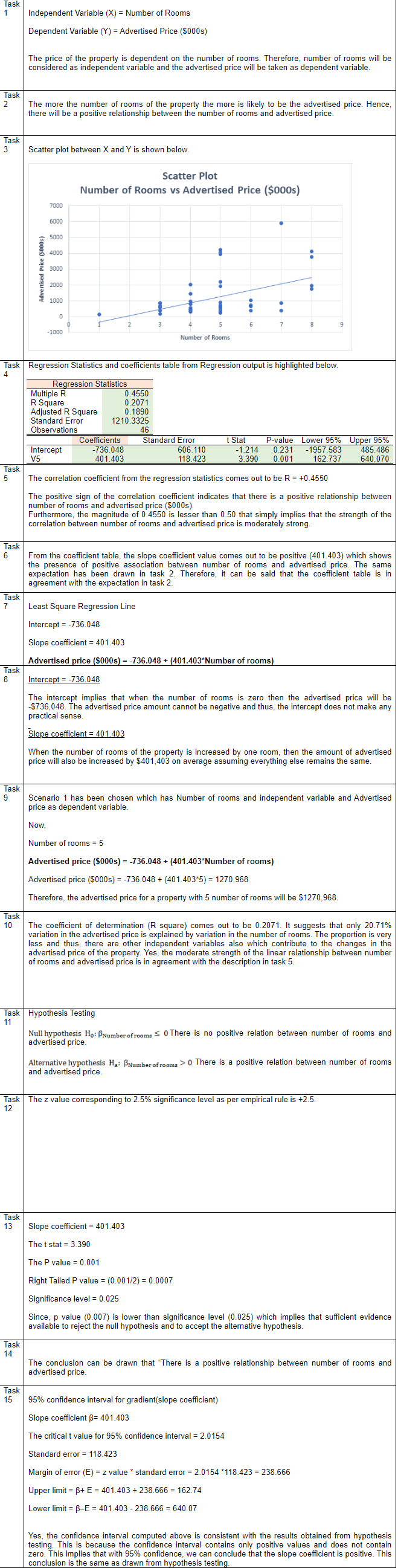## Statistical Analysis Assignment Sample

### INSTRUCTIONS

Perform the required calculations using Excel (and PHStat where appropriate), present your findings (i.e., the relevant output), and prepare short written responses to the following questions. Please note that you must provide a clear interpretation and explanation of the results reported in your output. Please submit your answers in a single Word file.

### Question 1 – Binomial Distribution (8 marks)

A university has found that 2.5% of its students withdraw without completing the introductory business analytics course. Assume that 100 students are registered for the course.

a) What is the probability that two or fewer students will withdraw?
b) What is the probability that exactly five students will withdraw?
c) What is the probability that more than three students will withdraw?
d) What is the expected number of withdrawals from this course?

### Question 2 – Normal Distribution

Suppose that the return for a particular investment is normally distributed with a population mean of 10.1% and a population standard deviation of 5.4%.
a) What is the probability that the investment has a return of at least 20%?
b) What is the probability that the investment has a return of 10% or less?
A person must score in the upper 5% of the population on an IQ test to qualify for a particular occupation.
c) If IQ scores are normally distributed with a mean of 100 and a standard deviation of 15, what score must a person have to qualify for this occupation

### Question 3 – Normal Distribution (4 marks)

According to a recent study, the average night’s sleep is 8 hours. Assume that the standard deviation is 1.1 hours and that the probability distribution is normal.
a) What is the probability that a randomly selected person sleeps for more than 8 hours?
b) Doctors suggest getting between 7 and 9 hours of sleep each night. What percentage of the population gets this much sleep?

### Question 4 – Normal Distribution (10 marks)

The time needed to complete a final examination in a particular college course is normally distributed with a mean of 160 minutes and a standard deviation of 25 minutes. Answer the following questions:

a) What is the probability of completing the exam in 120 minutes or less?
b) What is the probability that a student will complete the exam in more than 120 minutes but less than 150 minutes?
c) What is the probability that a student will complete the exam in more than 100 minutes but less than 170 minutes?
d) Assume that the class has 120 students and that the examination period is 180 minutes in length. How many students do you expect will not complete the examination in the allotted time?

## Solution

### Question 1

Here, number of trials (n) = 100
Probability of success i.e. student withdrawing from course (p) = 0.025
The various probabilities have been computed using BINOMIST function in Excel.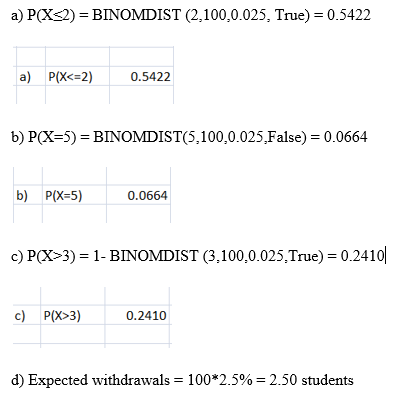### Question 2

a) Mean = 10.1%
Standard deviation = 5.4%
The relevant output from Excel is shown below.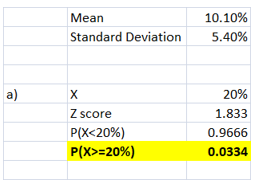The z score is computed using the inputs provided. Using the NORMS.DIST(1.833) function, the probability of P(X<20%) has been determined. Finally, the probability that the return would be atleast 20% is 0.0334.

b) Mean = 10.1%
Standard deviation = 5.4%
The relevant output from Excel is shown below for assignment help

The z score is computed using the inputs provided. Using the NORMS.DIST(-0.0185) function, the probability of P(X<10%) has been determined. It can be concluded that there is a probability of 0.4926 that the given investment has a return of 10% or less.

c) The relevant output from Excel is shown below.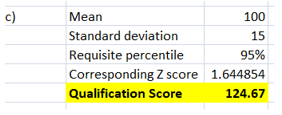The requisite percentile score required is 95%. The corresponding Z value for this as determined using NORMS.INV has come out as 1.644854. This along with the mean and standard deviation has been used to derive the minimum qualification score as 124.67. Hence, to be in the top 5%, a candidate needs to score atleast 124.67 in the IQ test.

### Question 3

a) The relevant output from Excel is shown below.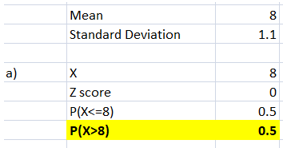Using the mean, standard deviation and the X value, the Z score has been computed as zero. Using NORMS.DIST(0) function, the probability of P(X<=8) has come out as 0.5. Further, the probability of P(X>8) has been computed as 0.5. Hence, the probability that a randomly selected person sleeps more than 8 hours is 0.5.

b) The relevant output from Excel is shown below.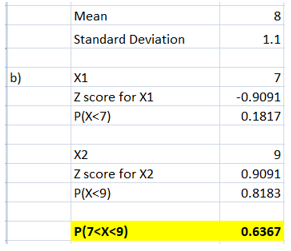For X1 = 7 hours, the corresponding z score was computed followed by finding the P(X<7) using NORMS.DIST(-0.9091) function. For X2 = 9 hours, the corresponding z score was computed followed by finding the P(X<9) using NORMS.DIST(0.9091) function. The probability that people would be getting sleep between 7 and 9 hours is 0.6367. Thus, 63.67% of the population is getting sleep between the 7 and 9 hours each night.

### Question 4

a) The relevant output from Excel is shown below.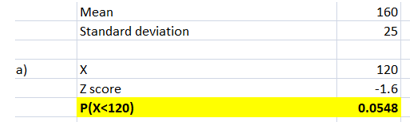For X =120, the corresponding z score was computed followed by finding the P(X<=120) using NORMS.DIST(-1.6) function. The probability of completing the exam in 120 minutes or lesser is 0.0548.

b) The relevant output from Excel is shown below.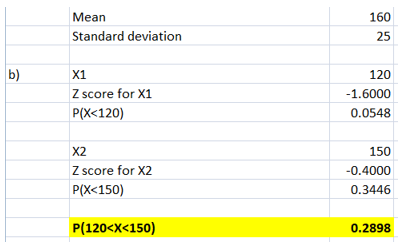For X1 = 120 minutes, the corresponding z score was computed followed by finding the P(X<120) using NORMS.DIST(-1.6) function. For X2 = 150 minutes, the corresponding z score was computed followed by finding the P(X< 150) using NORMS.DIST(-0.40) function. The probability that a student will complete the test in more than 120 minutes but less than 150 minutes is 0.2898.

c) The relevant output from Excel is shown below.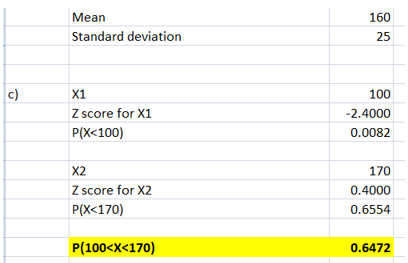For X1 = 100 minutes, the corresponding z score was computed followed by finding the P(X<100) using NORMS.DIST(-2.4) function. For X2 = 170 minutes, the corresponding z score was computed followed by finding the P(X< 170) using NORMS.DIST(0.40) function. The probability that a student will complete the test in more than 100 minutes but less than 170 minutes is 0.6472.

d) The relevant output from Excel is shown below.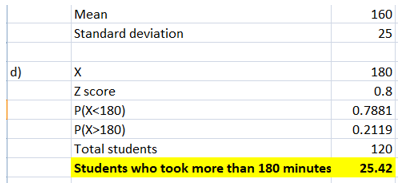For the score of 180 minutes, the corresponding Z score is 0.8. Using NORMS.DIST(0.8), the P(X<180) is 0.7881. Hence, the probability of student taking more than 180 minutes or not finishing within the allotted 180 minutes is 0.2119. The total number of students given is 120. Thus, students not finishing within the allotted 180 minutes is 0.2119*120 = 25.42 or 26 students.

## STATS7061 Statistical Analysis Assignment Sample

Prepare short written responses to the following questions. Please note that you must provide a clear interpretation and explanation of the results for assignment help reported in your output.

### Question 1 – Binomial Distribution

A university has found that 2.5% of its students withdraw without completing the introductory business analytics course. Assume that 100 students are registered for the course.

a) What is the probability that two or fewer students will withdraw?
b) What is the probability that exactly five students will withdraw?
c) What is the probability that more than three students will withdraw?
d) What is the expected number of withdrawals from this course?

### Question 1

Here, number of trials (n) = 100
Probability of success i.e. student withdrawing from course (p) = 0.025
The various probabilities have been computed using BINOMIST function in Excel.

a) P(X≤2) = BINOMDIST(2,100,0.025,True) = 0.5422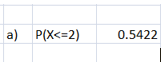b) P(X=5) = BINOMDIST(5,100,0.025,False) = 0.0664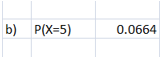c) P(X>3) = 1- BINOMDIST(3,100,0.025,True) = 0.2410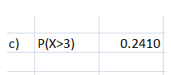d) Expected withdrawals = 100*2.5% = 2.50 students

### Question 2 – Normal Distribution

Suppose that the return for a particular investment is normally distributed with a population mean of 10.1% and a population standard deviation of 5.4%.

a) What is the probability that the investment has a return of at least 20%?

b) What is the probability that the investment has a return of 10% or less?
A person must score in the upper 5% of the population on an IQ test to qualify for a particular
occupation.

c) If IQ scores are normally distributed with a mean of 100 and a standard deviation of 15, what score must a person have to qualify for this occupation?

### Question 2

a) Mean = 10.1%
Standard deviation = 5.4%
The relevant output from Excel is shown below.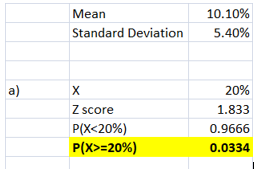The z score is computed using the inputs provided. Using the NORMS.DIST(1.833) function, the probability of P(X<20%) has been determined. Finally, the probability that the return would be atleast 20% is 0.0334.

b) Mean = 10.1%
Standard deviation = 5.4%
The relevant output from Excel is shown below.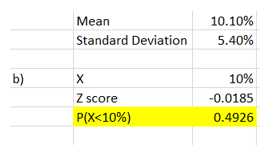The z score is computed using the inputs provided. Using the NORMS.DIST(-0.0185) function, the probability of P(X<10%) has been determined. It can be concluded that there is a probability of 0.4926 that the given investment has a return of 10% or less.

c) The relevant output from Excel is shown below.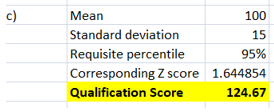The requisite percentile score required is 95%. The corresponding Z value for this as determined using NORMS.INV has come out as 1.644854. This along with the mean and standard deviation has been used to derive the minimum qualification score as 124.67. Hence, to be in the top 5%, a candidate needs to score atleast 124.67 in the IQ test.

### Question 3 – Normal Distribution

According to a recent study, the average night’s sleep is 8 hours. Assume that the standard deviation is 1.1 hours and that the probability distribution is normal.

a) What is the probability that a randomly selected person sleeps for more than 8 hours?
b) Doctors suggest getting between 7 and 9 hours of sleep each night. What percentage of the population gets this much sleep?

### Question 3

a) The relevant output from Excel is shown below.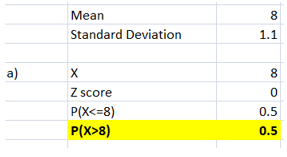Using the mean, standard deviation and the X value, the Z score has been computed as zero. Using NORMS.DIST(0) function, the probability of P(X<=8) has come out as 0.5. Further, the probability of P(X>8) has been computed as 0.5. Hence, the probability that a randomly selected person sleeps more than 8 hours is 0.5.

b) The relevant output from Excel is shown below.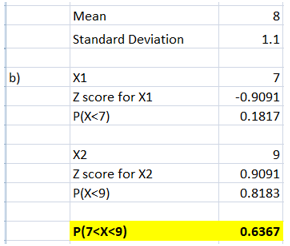For X1 = 7 hours, the corresponding z score was computed followed by finding the P(X<7) using NORMS.DIST(-0.9091) function. For X2 = 9 hours, the corresponding z score was computed followed by finding the P(X<9) using NORMS.DIST(0.9091) function. The probability that people would be getting sleep between 7 and 9 hours is 0.6367. Thus, 63.67% of the population is getting sleep between the 7 and 9 hours each night.

### Question 4 – Normal Distribution

The time needed to complete a final examination in a particular college course is normally distributed with a mean of 160 minutes and a standard deviation of 25 minutes. Answer the following questions:

a) What is the probability of completing the exam in 120 minutes or less?

b) What is the probability that a student will complete the exam in more than 120 minutes but less than 150 minutes?

c) What is the probability that a student will complete the exam in more than 100 minutes but less than 170 minutes?

d) Assume that the class has 120 students and that the examination period is 180 minutes in length. How many students do you expect will not complete the examination in the allotted time?

### Question 4

a) The relevant output from Excel is shown below.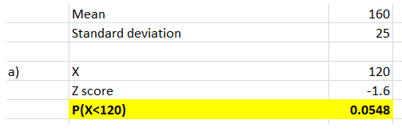For X =120, the corresponding z score was computed followed by finding the P(X<=120) using NORMS.DIST(-1.6) function. The probability of completing the exam in 120 minutes or lesser is 0.0548.

b) The relevant output from Excel is shown below.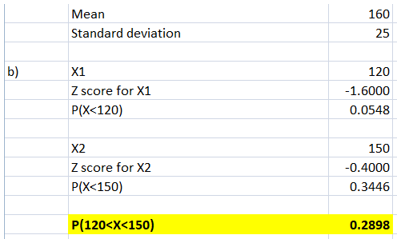For X1 = 120 minutes, the corresponding z score was computed followed by finding the P(X<120) using NORMS.DIST(-1.6) function. For X2 = 150 minutes, the corresponding z score was computed followed by finding the P(X< 150) using NORMS.DIST(-0.40) function. The probability that a student will complete the test in more than 120 minutes but less than 150 minutes is 0.2898.

c) The relevant output from Excel is shown below.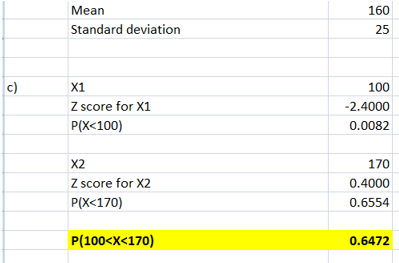For X1 = 100 minutes, the corresponding z score was computed followed by finding the P(X<100) using NORMS.DIST(-2.4) function. For X2 = 170 minutes, the corresponding z score was computed followed by finding the P(X< 170) using NORMS.DIST(0.40) function. The probability that a student will complete the test in more than 100 minutes but less than 170 minutes is 0.6472.

d) The relevant output from Excel is shown below.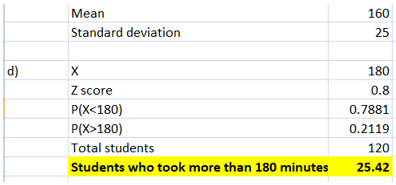For the score of 180 minutes, the corresponding Z score is 0.8. Using NORMS.DIST(0.8), the P(X<180) is 0.7881. Hence, the probability of student taking more than 180 minutes or not finishing within the allotted 180 minutes is 0.2119. The total number of students given is 120. Thus, students not finishing within the allotted 180 minutes is 0.2119*120 = 25.42 or 26 students.